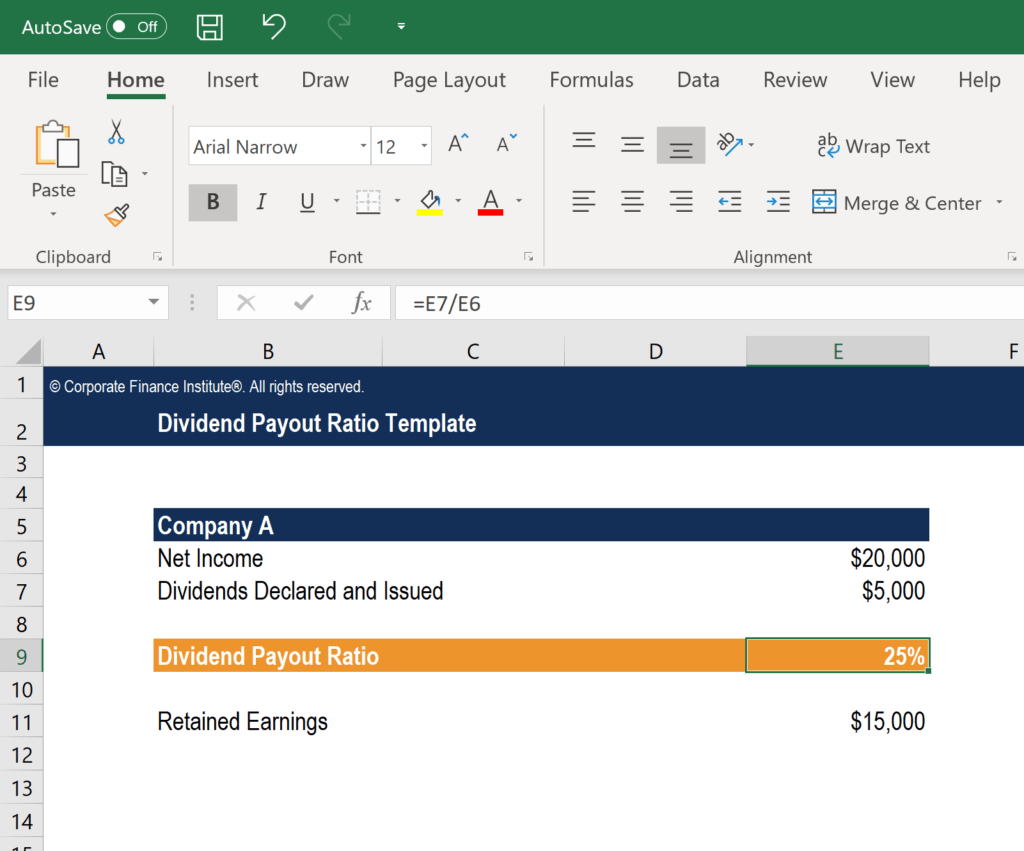# Dividend Payout Ratio Template

## Dividend Payout Ratio Template

This Dividend Payout Ratio Template will show you how to calculate the dividend payout ratio using the formula: DPR = Total dividends / Net income.

Here is a preview of the template:### What is Dividend Payout Ratio (DPR)?

The Dividend Payout Ratio (DPR) is the amount of dividends paid to shareholders in relation to the total amount of net income the company generates. In other words, the dividend payout ratio measures the percentage of net income that is distributed to shareholders in the form of dividends.

### Dividend Payout Ratio Formula

There are several formulas for calculating DPR:

1. DPR = Total dividends / Net income

2. DPR = 1 – Retention ratio (the retention ratio, which measures the percentage of net income that is kept by the company as retained earnings, is the opposite, or inverse, of the dividend payout ratio

3. DPR = Dividends per share / Earnings per share

More Free Templates

For more resources, check out our business templates library to download numerous free Excel modeling, PowerPoint presentation and Word document templates.

• Excel Modeling Templates
• PowerPoint Presentation Templates
• Transaction Document Templates

### Financial Analyst Certification

Become a certified Financial Modeling and Valuation Analyst (FMVA)® by completing CFI’s online financial modeling classes and training program!